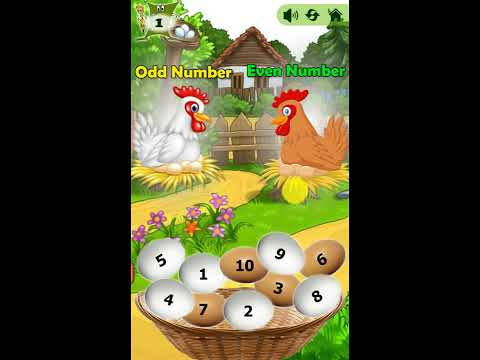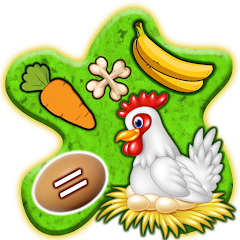# Math Drills Educational Game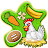50+Everyone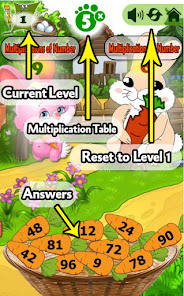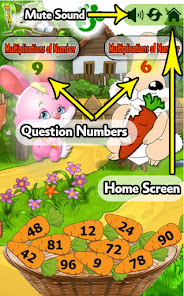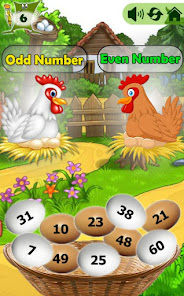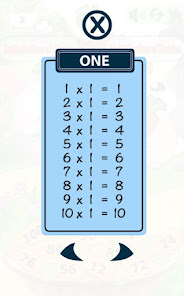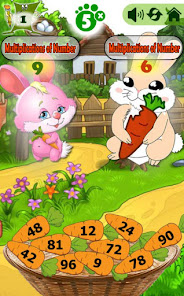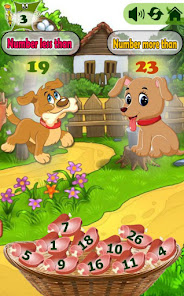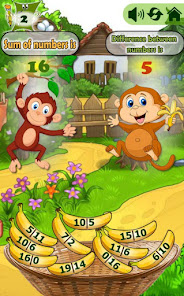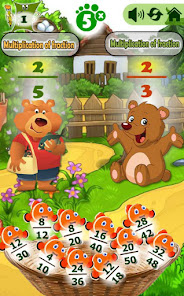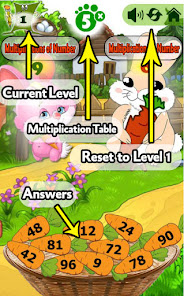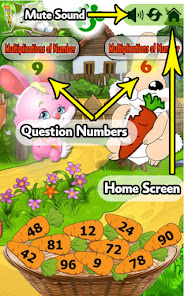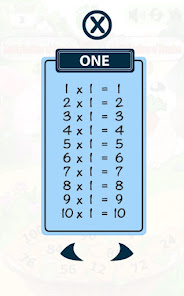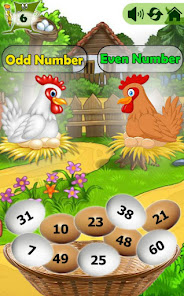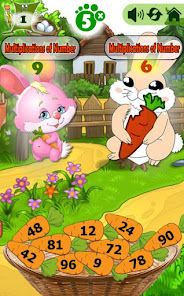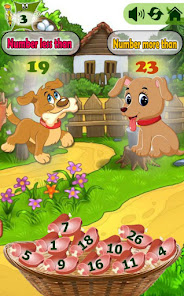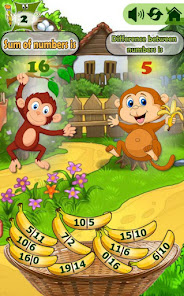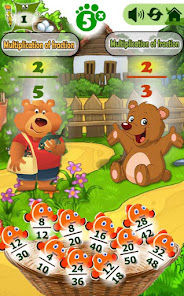The Math Exercises App (In English and Arabic) is one of the best educational apps that offer a group of math questions that are repeated in many curriculum and exams. Examples of our math games: multiplying fractions, adding and subtracting integers, multiplication practice, and math drills multiplication with multiplication table. 1️⃣2️⃣3️⃣🔢
👉 The app can generate endless random math drills, math worksheets grade 1 📃, math worksheets for grade 2 📰, math worksheets grade 3 📑, math worksheets grade 4 📜, math worksheets grade 5 📄, math worksheets grade 7, maths questions for 7 year olds, mathworksheets4kids, numbers worksheet, free math worksheets, or math practice test.
It is possible to print out math practice worksheets in a very beautiful motivating kiddy math practice worksheets.
👉 Teachers can use it to automatically generate sample basic math test for employment.
Mathematics is considered by many kids as difficult because it is not presented in a funny way as it should be.
👉 This is why we have provided you with this math App to raise the level of interesting in learning snappy maths by supporting colors, images, and sounds that attract children to use mobile phones and tablets in a meaningful manner.
👉 This educational apps currently offers five types of drills, each of which generates an infinite number of questions randomly. Whenever the child answers a question he will be moved to a higher level which contains more difficult numbers and equations. Also, there is an icon shows times tables.

👉 Math drills and math questions are as follows: 👇👇
1 - Specification of the even and odd numbers: Here App will display for kids ten numbers without repetition randomly distributed in the form of eggs in a large basket and the user has to distribute these eggs to two opposite hens, one represents the even number and the other represents the odd number with titles above both.

2 - Numbers Multiplications (math drills multiplication): in which the application displays ten numbers randomly each in a carrot, and there are two rabbits with the title "Multiplications of number X", the child should move each carrot to the appropriate rabbit according to the number displayed above the rabbit, with multiplication table and times table chart.

3 - Comparisons: In this exercise, the program produces ten random numbers, each on a small piece of meat. The player must distribute these numbers of meat pieces between two dogs. The first represents the set of numbers larger than a certain number and the second represents a set of numbers smaller than a dedicated number. The child should identify the right dog to be fed by a piece of meat carried by the child's finger.

4 - Addition Subtraction: In this exercise we show two beautiful monkeys, one of them has the title "Sum of numbers is XX" and the other's title is "Difference between numbers is XX". There is also a group of bananas inside the basket, each of which has two numbers and the child must determine for which monkey it is supposed to move each banana.

5 - Fraction multiplications (multiplying fractions): In this exercise, two beautiful fat bears are there. Each of them has the title "Multiplication of fraction", and there is also a group of simple fractions each of which is written on a fish. The child has to determine for which bear it's supposed to move each Fish. In this exercise, the student attempts to identify equal fractions between bears and fish and solve multiplying fractions, with a multiplication table.

We can print multiplication worksheets and fractions worksheets using exercises 2 and 5, addition and subtraction worksheets, addition worksheets using exercise 4, and kindergarten math worksheets using test 1.
You can open a multiplication chart showing each table on one page.

In the future, we are going to add math salamanders and multiply fractions by whole numbers.
Updated on
Apr 17, 2021

## Data safety

Safety starts with understanding how developers collect and share your data. Data privacy and security practices may vary based on your use, region, and age. The developer provided this information and may update it over time.No data shared with third parties